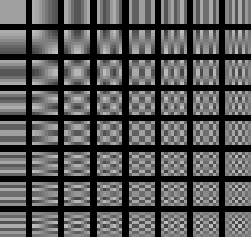Main Content

## Discrete Cosine Transform

### DCT Definition

The discrete cosine transform (DCT) represents an image as a sum of sinusoids of varying magnitudes and frequencies. The `dct2` function computes the two-dimensional discrete cosine transform (DCT) of an image. The DCT has the property that, for a typical image, most of the visually significant information about the image is concentrated in just a few coefficients of the DCT. For this reason, the DCT is often used in image compression applications. For example, the DCT is at the heart of the international standard lossy image compression algorithm known as JPEG. (The name comes from the working group that developed the standard: the Joint Photographic Experts Group.)

The two-dimensional DCT of an M-by-N matrix `A` is defined as follows.

`$\begin{array}{l}\begin{array}{cc}{B}_{pq}={\alpha }_{p}{\alpha }_{q}\sum _{m=0}^{M-1}\sum _{n=0}^{N-1}{A}_{mn}\mathrm{cos}\frac{\pi \left(2m+1\right)p}{2M}\mathrm{cos}\frac{\pi \left(2n+1\right)q}{2N},& \begin{array}{l}0\le p\le M-1\\ 0\le q\le N-1\end{array}\end{array}\\ \\ \begin{array}{cccc}{\alpha }_{p}=\left\{\begin{array}{l}1/\sqrt{M},\\ \sqrt{2/M},\end{array}& \begin{array}{l}p=0\\ 1\le p\le M-1\end{array}& {\alpha }_{q}=\left\{\begin{array}{l}1/\sqrt{N},\\ \sqrt{2/N},\end{array}& \begin{array}{l}q=0\\ 1\le q\le N-1\end{array}\end{array}\end{array}$`

The values Bpq are called the DCT coefficients of `A`. (Note that matrix indices in MATLAB® always start at 1 rather than 0; therefore, the MATLAB matrix elements `A(1,1)` and `B(1,1)` correspond to the mathematical quantities A00 and B00, respectively.)

The DCT is an invertible transform, and its inverse is given by

`$\begin{array}{l}\begin{array}{cc}{A}_{mn}=\sum _{p=0}^{M-1}\sum _{q=0}^{N-1}{\alpha }_{p}{\alpha }_{q}{B}_{pq}\mathrm{cos}\frac{\pi \left(2m+1\right)p}{2M}\mathrm{cos}\frac{\pi \left(2n+1\right)q}{2N},& \begin{array}{l}0\le m\le M-1\\ 0\le n\le N-1\end{array}\end{array}\\ \\ \begin{array}{cccc}{\alpha }_{p}=\left\{\begin{array}{l}1/\sqrt{M},\\ \sqrt{2/M},\end{array}& \begin{array}{l}p=0\\ 1\le p\le M-1\end{array}& {\alpha }_{q}=\left\{\begin{array}{l}1/\sqrt{N},\\ \sqrt{2/N},\end{array}& \begin{array}{l}q=0\\ 1\le q\le N-1\end{array}\end{array}\end{array}$`

The inverse DCT equation can be interpreted as meaning that any M-by-N matrix `A` can be written as a sum of MN functions of the form

These functions are called the basis functions of the DCT. The DCT coefficients Bpq, then, can be regarded as the weights applied to each basis function. For 8-by-8 matrices, the 64 basis functions are illustrated by this image.

The 64 Basis Functions of an 8-by-8 MatrixHorizontal frequencies increase from left to right, and vertical frequencies increase from top to bottom. The constant-valued basis function at the upper left is often called the DC basis function, and the corresponding DCT coefficient B00 is often called the DC coefficient.

### The DCT Transform Matrix

There are two ways to compute the DCT using Image Processing Toolbox™ software. The first method is to use the `dct2` function. `dct2` uses an FFT-based algorithm for speedy computation with large inputs. The second method is to use the DCT transform matrix, which is returned by the function `dctmtx` and might be more efficient for small square inputs, such as 8-by-8 or 16-by-16. The M-by-M transform matrix `T` is given by

`$\begin{array}{ccc}{T}_{pq}=\left\{\begin{array}{l}\frac{1}{\sqrt{M}}\\ \sqrt{\frac{2}{M}}\mathrm{cos}\frac{\pi \left(2q+1\right)p}{2M}\end{array}& \begin{array}{l}p=0,\\ \\ 1\le p\le M-1,\end{array}& \begin{array}{l}0\le q\le M-1\\ \\ 0\le q\le M-1\end{array}\end{array}$`

For an M-by-M matrix `A`, `T*A` is an M-by-M matrix whose columns contain the one-dimensional DCT of the columns of `A`. The two-dimensional DCT of `A` can be computed as `B=T*A*T'`. Since `T` is a real orthonormal matrix, its inverse is the same as its transpose. Therefore, the inverse two-dimensional DCT of `B` is given by `T'*B*T`.

### Image Compression with the Discrete Cosine Transform

This example shows how to compress an image using the Discrete Cosine Transform (DCT). The example computes the two-dimensional DCT of 8-by-8 blocks in an input image, discards (sets to zero) all but 10 of the 64 DCT coefficients in each block, and then reconstructs the image using the two-dimensional inverse DCT of each block. The example uses the transform matrix computation method.

DCT is used in the JPEG image compression algorithm. The input image is divided into 8-by-8 or 16-by-16 blocks, and the two-dimensional DCT is computed for each block. The DCT coefficients are then quantized, coded, and transmitted. The JPEG receiver (or JPEG file reader) decodes the quantized DCT coefficients, computes the inverse two-dimensional DCT of each block, and then puts the blocks back together into a single image. For typical images, many of the DCT coefficients have values close to zero. These coefficients can be discarded without seriously affecting the quality of the reconstructed image.

Read an image into the workspace and convert it to class `double`.

```I = imread('cameraman.tif'); I = im2double(I);```

Compute the two-dimensional DCT of 8-by-8 blocks in the image. The function `dctmtx` returns the N-by-N DCT transform matrix.

```T = dctmtx(8); dct = @(block_struct) T * block_struct.data * T'; B = blockproc(I,[8 8],dct);```

Discard all but 10 of the 64 DCT coefficients in each block.

```mask = [1 1 1 1 0 0 0 0 1 1 1 0 0 0 0 0 1 1 0 0 0 0 0 0 1 0 0 0 0 0 0 0 0 0 0 0 0 0 0 0 0 0 0 0 0 0 0 0 0 0 0 0 0 0 0 0 0 0 0 0 0 0 0 0]; B2 = blockproc(B,[8 8],@(block_struct) mask .* block_struct.data);```

Reconstruct the image using the two-dimensional inverse DCT of each block.

```invdct = @(block_struct) T' * block_struct.data * T; I2 = blockproc(B2,[8 8],invdct);```

Display the original image and the reconstructed image, side-by-side. Although there is some loss of quality in the reconstructed image, it is clearly recognizable, even though almost 85% of the DCT coefficients were discarded.

`imshow(I)````figure imshow(I2)```Download ebook